Next: Charged Particle Motion in Up: Quantum Dynamics Previous: Ehrenfest Theorem

# Schrödinger Wave Equation

Consider the motion of a particle in three dimensions in the Schrödinger picture. The fixed dynamical variables of the system are the position operators,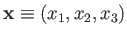, and the momentum operators,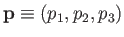. The state of the system is represented as some time evolving ket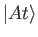.

Let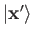represent a simultaneous eigenket of the position operators belonging to the eigenvalues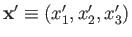. Note that, because the position operators are fixed in the Schrödinger picture, we do not expect theto evolve in time. The wavefunction of the system at time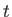is defined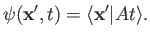(3.48)

The Hamiltonian of the system is taken to be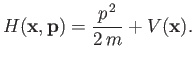(3.49)

The Schrödinger equation of motion, (3.10), yields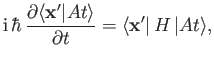(3.50)

where use has been made of the time independence of the. We adopt the Schrödinger representation in which the momentum conjugate to the position operator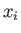is written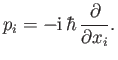(3.51)

[See Equation (2.74).] Thus,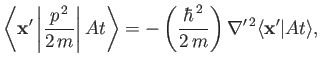(3.52)

where use has been made of Equation (2.78). Here,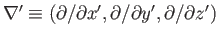denotes the gradient operator written in terms of the position eigenvalues. We can also write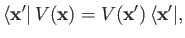(3.53)

where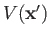is a scalar function of the position eigenvalues. Combining Equations (3.49), (3.50), (3.52), and (3.53), we obtain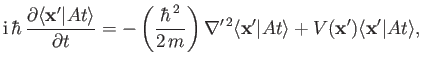(3.54)

which can also be written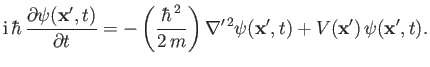(3.55)

This is the Schrödinger time-dependent wave equation, and is the basis of wave mechanics . Note, however, that the wave equation is just one of many possible representations of quantum mechanics. It just happens to give a type of equation that we know how to solve. In deriving the wave equation, we have chosen to represent the system in terms of the eigenkets of the position operators, instead of those of the momentum operators. We have also fixed the relative phases of theaccording to the Schrödinger representation, so that Equation (3.51) is valid. Finally, we have chosen to work in the Schrödinger picture, in which state kets evolve and dynamical variables are fixed, instead of the Heisenberg picture, in which the opposite is true.

Suppose that the ketis an eigenket of the Hamiltonian belonging to the eigenvalue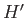: that is,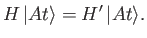(3.56)

The Schrödinger equation of motion, (3.10), yields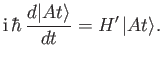(3.57)

This can be integrated to give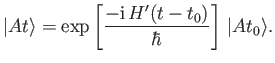(3.58)

Note thatonly differs from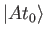by a phase-factor. The direction of the vector remains fixed in ket space. This suggests that if the system is initially in an eigenstate of the Hamiltonian then it remains in this state for ever, as long as the system is undisturbed. Such a state is called a stationary state. The wavefunction of a stationary state satisfies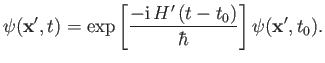(3.59)

Substituting the previous relation into the Schrödinger time-dependent wave equation, (3.55), we obtain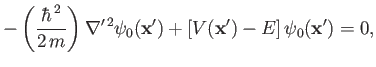(3.60)

where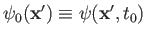, and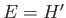is the energy of the system. This is the Schrödinger time-independent wave equation. A bound state solution of the previous equation, in which the particle is confined within a finite region of space, satisfies the boundary condition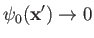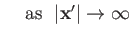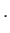(3.61)

Such a solution is only possible if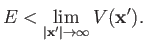(3.62)

Because it is conventional to set the potential at infinity equal to zero, the previous relation implies that bound states are equivalent to negative energy states . The boundary condition (3.61) is sufficient to uniquely specify the solution of Equation (3.60).

The quantity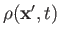, defined by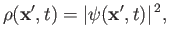(3.63)

is termed the probability density. Recall, from a direct generalization of Equation (2.30), that the probability of observing the particle in some volume element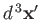around position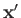is proportional to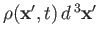. The probability is equal toif the wavefunction is properly normalized, so that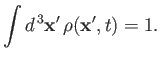(3.64)

The Schrödinger time-dependent wave equation, (3.55), can easily be transformed into a conservation equation for the probability density: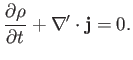(3.65)

The probability current,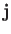, takes the form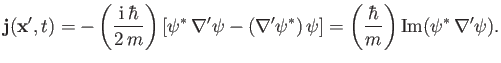(3.66)

We can integrate Equation (3.65) over all space, using the divergence theorem , and the boundary condition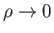as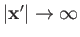, to obtain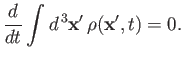(3.67)

Thus, the Schrödinger time-dependent wave equation conserves probability. In particular, if the wavefunction starts off properly normalized, according to Equation (3.64), then it remains properly normalized at all subsequent times. It is easily demonstrated that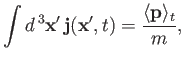(3.68)

where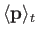denotes the expectation value of the momentum evaluated at time. Clearly, the probability current is indirectly related to the particle momentum.

In deriving Equations (3.65), we have, naturally, assumed that the potential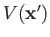is real. Suppose, however, that the potential has an imaginary component. In this case, Equation (3.65) generalizes to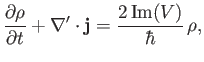(3.69)

giving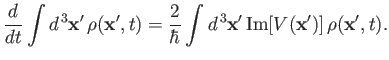(3.70)

(See Exercise 2.) Thus, if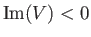then the total probability of observing the particle anywhere in space decreases monotonically with time. Hence, an imaginary potential can be used to account for the disappearance or decay of a particle. Such a potential is often employed to model nuclear reactions in which incident particles are absorbed by nuclei.Next: Charged Particle Motion in Up: Quantum Dynamics Previous: Ehrenfest Theorem
Richard Fitzpatrick 2016-01-22